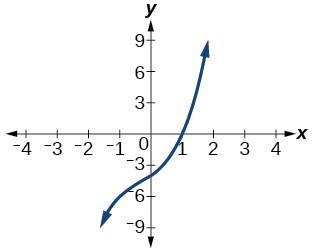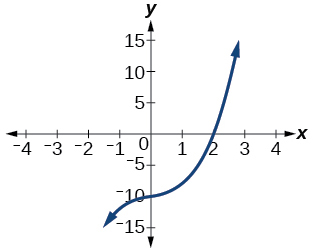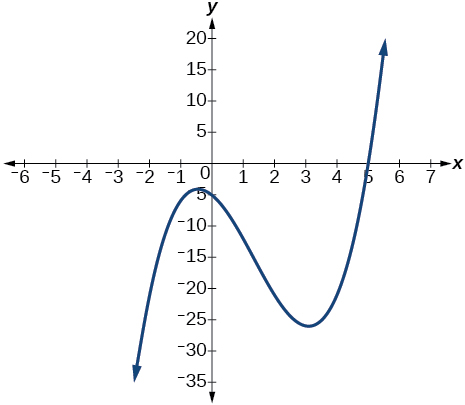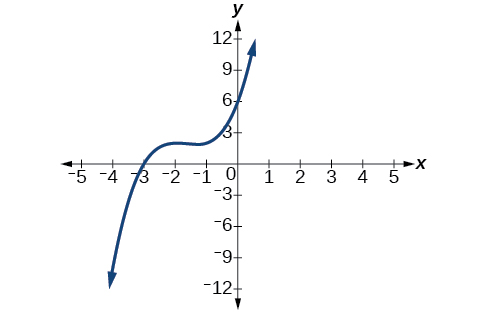# 5.4 Dividing polynomials  (Page 4/6)

 Page 4 / 6

## Verbal

If division of a polynomial by a binomial results in a remainder of zero, what can be conclude?

The binomial is a factor of the polynomial.

If a polynomial of degree $\text{\hspace{0.17em}}n\text{\hspace{0.17em}}$ is divided by a binomial of degree 1, what is the degree of the quotient?

## Algebraic

For the following exercises, use long division to divide. Specify the quotient and the remainder.

$\left({x}^{2}+5x-1\right)÷\left(x-1\right)$

$x+6+\frac{5}{x-1}\text{,}\text{\hspace{0.17em}}\text{quotient:}\text{\hspace{0.17em}}x+6\text{,}\text{\hspace{0.17em}}\text{remainder:}\text{\hspace{0.17em}}\text{5}$

$\left(2{x}^{2}-9x-5\right)÷\left(x-5\right)$

$\left(3{x}^{2}+23x+14\right)÷\left(x+7\right)$

$\left(4{x}^{2}-10x+6\right)÷\left(4x+2\right)$

$\left(6{x}^{2}-25x-25\right)÷\left(6x+5\right)$

$x-5\text{,}\text{\hspace{0.17em}}\text{quotient:}\text{\hspace{0.17em}}x-5\text{,}\text{\hspace{0.17em}}\text{remainder:}\text{\hspace{0.17em}}\text{0}$

$\left(-{x}^{2}-1\right)÷\left(x+1\right)$

$\left(2{x}^{2}-3x+2\right)÷\left(x+2\right)$

$2x-7+\frac{16}{x+2}\text{,}\text{\hspace{0.17em}}\text{quotient:}\text{​}\text{\hspace{0.17em}}2x-7\text{,}\text{\hspace{0.17em}}\text{remainder:}\text{\hspace{0.17em}}\text{16}$

$\left({x}^{3}-126\right)÷\left(x-5\right)$

$\left(3{x}^{2}-5x+4\right)÷\left(3x+1\right)$

$x-2+\frac{6}{3x+1}\text{,}\text{\hspace{0.17em}}\text{quotient:}\text{\hspace{0.17em}}x-2\text{,}\text{\hspace{0.17em}}\text{remainder:}\text{\hspace{0.17em}}\text{6}$

$\left({x}^{3}-3{x}^{2}+5x-6\right)÷\left(x-2\right)$

$\left(2{x}^{3}+3{x}^{2}-4x+15\right)÷\left(x+3\right)$

$2{x}^{2}-3x+5\text{,}\text{\hspace{0.17em}}\text{quotient:}\text{\hspace{0.17em}}2{x}^{2}-3x+5\text{,}\text{\hspace{0.17em}}\text{remainder:}\text{\hspace{0.17em}}\text{0}$

For the following exercises, use synthetic division to find the quotient.

$\left(3{x}^{3}-2{x}^{2}+x-4\right)÷\left(x+3\right)$

$\left(2{x}^{3}-6{x}^{2}-7x+6\right)÷\left(x-4\right)$

$2{x}^{2}+2x+1+\frac{10}{x-4}$

$\left(6{x}^{3}-10{x}^{2}-7x-15\right)÷\left(x+1\right)$

$\left(4{x}^{3}-12{x}^{2}-5x-1\right)÷\left(2x+1\right)$

$2{x}^{2}-7x+1-\frac{2}{2x+1}$

$\left(9{x}^{3}-9{x}^{2}+18x+5\right)÷\left(3x-1\right)$

$\left(3{x}^{3}-2{x}^{2}+x-4\right)÷\left(x+3\right)$

$3{x}^{2}-11x+34-\frac{106}{x+3}$

$\left(-6{x}^{3}+{x}^{2}-4\right)÷\left(2x-3\right)$

$\left(2{x}^{3}+7{x}^{2}-13x-3\right)÷\left(2x-3\right)$

${x}^{2}+5x+1$

$\left(3{x}^{3}-5{x}^{2}+2x+3\right)÷\left(x+2\right)$

$\left(4{x}^{3}-5{x}^{2}+13\right)÷\left(x+4\right)$

$4{x}^{2}-21x+84-\frac{323}{x+4}$

$\left({x}^{3}-3x+2\right)÷\left(x+2\right)$

$\left({x}^{3}-21{x}^{2}+147x-343\right)÷\left(x-7\right)$

${x}^{2}-14x+49$

$\left({x}^{3}-15{x}^{2}+75x-125\right)÷\left(x-5\right)$

$\left(9{x}^{3}-x+2\right)÷\left(3x-1\right)$

$3{x}^{2}+x+\frac{2}{3x-1}$

$\left(6{x}^{3}-{x}^{2}+5x+2\right)÷\left(3x+1\right)$

$\left({x}^{4}+{x}^{3}-3{x}^{2}-2x+1\right)÷\left(x+1\right)$

${x}^{3}-3x+1$

$\left({x}^{4}-3{x}^{2}+1\right)÷\left(x-1\right)$

$\left({x}^{4}+2{x}^{3}-3{x}^{2}+2x+6\right)÷\left(x+3\right)$

${x}^{3}-{x}^{2}+2$

$\left({x}^{4}-10{x}^{3}+37{x}^{2}-60x+36\right)÷\left(x-2\right)$

$\left({x}^{4}-8{x}^{3}+24{x}^{2}-32x+16\right)÷\left(x-2\right)$

${x}^{3}-6{x}^{2}+12x-8$

$\left({x}^{4}+5{x}^{3}-3{x}^{2}-13x+10\right)÷\left(x+5\right)$

$\left({x}^{4}-12{x}^{3}+54{x}^{2}-108x+81\right)÷\left(x-3\right)$

${x}^{3}-9{x}^{2}+27x-27$

$\left(4{x}^{4}-2{x}^{3}-4x+2\right)÷\left(2x-1\right)$

$\left(4{x}^{4}+2{x}^{3}-4{x}^{2}+2x+2\right)÷\left(2x+1\right)$

$2{x}^{3}-2x+2$

For the following exercises, use synthetic division to determine whether the first expression is a factor of the second. If it is, indicate the factorization.

$x-2,\text{\hspace{0.17em}}4{x}^{3}-3{x}^{2}-8x+4$

$x-2,\text{\hspace{0.17em}}3{x}^{4}-6{x}^{3}-5x+10$

Yes $\text{\hspace{0.17em}}\left(x-2\right)\left(3{x}^{3}-5\right)$

$x+3,\text{\hspace{0.17em}}-4{x}^{3}+5{x}^{2}+8$

$x-2,\text{\hspace{0.17em}}4{x}^{4}-15{x}^{2}-4$

Yes $\text{\hspace{0.17em}}\left(x-2\right)\left(4{x}^{3}+8{x}^{2}+x+2\right)$

$x-\frac{1}{2},\text{\hspace{0.17em}}2{x}^{4}-{x}^{3}+2x-1$

$x+\frac{1}{3},\text{\hspace{0.17em}}3{x}^{4}+{x}^{3}-3x+1$

No

## Graphical

For the following exercises, use the graph of the third-degree polynomial and one factor to write the factored form of the polynomial suggested by the graph. The leading coefficient is one.

Factor is $\text{\hspace{0.17em}}{x}^{2}-x+3$Factor is $\text{\hspace{0.17em}}\left({x}^{2}+2x+4\right)$$\left(x-1\right)\left({x}^{2}+2x+4\right)$

Factor is $\text{\hspace{0.17em}}{x}^{2}+2x+5$Factor is $\text{\hspace{0.17em}}{x}^{2}+x+1$$\left(x-5\right)\left({x}^{2}+x+1\right)$

Factor is ${x}^{2}+2x+2$For the following exercises, use synthetic division to find the quotient and remainder.

$\frac{4{x}^{3}-33}{x-2}$

$\text{Quotient:}\text{\hspace{0.17em}}4{x}^{2}+8x+16\text{,}\text{\hspace{0.17em}}\text{remainder:}\text{\hspace{0.17em}}-1$

$\frac{2{x}^{3}+25}{x+3}$

$\frac{3{x}^{3}+2x-5}{x-1}$

$\text{Quotient:}\text{\hspace{0.17em}}3{x}^{2}+3x+5\text{,}\text{\hspace{0.17em}}\text{remainder:}\text{\hspace{0.17em}}0$

$\frac{-4{x}^{3}-{x}^{2}-12}{x+4}$

$\frac{{x}^{4}-22}{x+2}$

$\text{Quotient:}\text{\hspace{0.17em}}{x}^{3}-2{x}^{2}+4x-8\text{,}\text{\hspace{0.17em}}\text{remainder:}\text{\hspace{0.17em}}-6$

## Technology

For the following exercises, use a calculator with CAS to answer the questions.

Consider $\text{\hspace{0.17em}}\frac{{x}^{k}-1}{x-1}\text{\hspace{0.17em}}$ with What do you expect the result to be if $\text{\hspace{0.17em}}k=4?$

Consider $\text{\hspace{0.17em}}\frac{{x}^{k}+1}{x+1}\text{\hspace{0.17em}}$ for What do you expect the result to be if $\text{\hspace{0.17em}}k=7?$

${x}^{6}-{x}^{5}+{x}^{4}-{x}^{3}+{x}^{2}-x+1$

Consider $\text{\hspace{0.17em}}\frac{{x}^{4}-{k}^{4}}{x-k}\text{\hspace{0.17em}}$ for What do you expect the result to be if $\text{\hspace{0.17em}}k=4?$

Consider $\text{\hspace{0.17em}}\frac{{x}^{k}}{x+1}\text{\hspace{0.17em}}$ with What do you expect the result to be if $\text{\hspace{0.17em}}k=4?$

${x}^{3}-{x}^{2}+x-1+\frac{1}{x+1}$

Consider $\text{\hspace{0.17em}}\frac{{x}^{k}}{x-1}\text{\hspace{0.17em}}$ with What do you expect the result to be if $\text{\hspace{0.17em}}k=4?$

## Extensions

For the following exercises, use synthetic division to determine the quotient involving a complex number.

$\frac{x+1}{x-i}$

$1+\frac{1+i}{x-i}$

$\frac{{x}^{2}+1}{x-i}$

$\frac{x+1}{x+i}$

$1+\frac{1-i}{x+i}$

$\frac{{x}^{2}+1}{x+i}$

$\frac{{x}^{3}+1}{x-i}$

${x}^{2}-ix-1+\frac{1-i}{x-i}$

## Real-world applications

For the following exercises, use the given length and area of a rectangle to express the width algebraically.

Length is $\text{\hspace{0.17em}}x+5,\text{\hspace{0.17em}}$ area is $\text{\hspace{0.17em}}2{x}^{2}+9x-5.$

Length is area is $\text{\hspace{0.17em}}4{x}^{3}+10{x}^{2}+6x+15$

$2{x}^{2}+3$

Length is $\text{\hspace{0.17em}}3x–4,\text{\hspace{0.17em}}$ area is $\text{\hspace{0.17em}}6{x}^{4}-8{x}^{3}+9{x}^{2}-9x-4$

For the following exercises, use the given volume of a box and its length and width to express the height of the box algebraically.

Volume is $\text{\hspace{0.17em}}12{x}^{3}+20{x}^{2}-21x-36,\text{\hspace{0.17em}}$ length is $\text{\hspace{0.17em}}2x+3,\text{\hspace{0.17em}}$ width is $\text{\hspace{0.17em}}3x-4.$

$2x+3$

Volume is $\text{\hspace{0.17em}}18{x}^{3}-21{x}^{2}-40x+48,\text{\hspace{0.17em}}$ length is $\text{\hspace{0.17em}}3x–4,\text{\hspace{0.17em}}$ width is $\text{\hspace{0.17em}}3x–4.$

Volume is $\text{\hspace{0.17em}}10{x}^{3}+27{x}^{2}+2x-24,\text{\hspace{0.17em}}$ length is $\text{\hspace{0.17em}}5x–4,\text{\hspace{0.17em}}$ width is $\text{\hspace{0.17em}}2x+3.$

$x+2$

Volume is $\text{\hspace{0.17em}}10{x}^{3}+30{x}^{2}-8x-24,\text{\hspace{0.17em}}$ length is $\text{\hspace{0.17em}}2,\text{\hspace{0.17em}}$ width is $\text{\hspace{0.17em}}x+3.$

For the following exercises, use the given volume and radius of a cylinder to express the height of the cylinder algebraically.

Volume is $\text{\hspace{0.17em}}\pi \left(25{x}^{3}-65{x}^{2}-29x-3\right),\text{\hspace{0.17em}}$ radius is $\text{\hspace{0.17em}}5x+1.$

$x-3$

Volume is $\text{\hspace{0.17em}}\pi \left(4{x}^{3}+12{x}^{2}-15x-50\right),\text{\hspace{0.17em}}$ radius is $\text{\hspace{0.17em}}2x+5.$

Volume is $\text{\hspace{0.17em}}\pi \left(3{x}^{4}+24{x}^{3}+46{x}^{2}-16x-32\right),\text{\hspace{0.17em}}$ radius is $\text{\hspace{0.17em}}x+4.$

$3{x}^{2}-2$

bsc F. y algebra and trigonometry pepper 2
given that x= 3/5 find sin 3x
4
DB
remove any signs and collect terms of -2(8a-3b-c)
-16a+6b+2c
Will
Joeval
(x2-2x+8)-4(x2-3x+5)
sorry
Miranda
x²-2x+9-4x²+12x-20 -3x²+10x+11
Miranda
x²-2x+9-4x²+12x-20 -3x²+10x+11
Miranda
(X2-2X+8)-4(X2-3X+5)=0 ?
master
The anwser is imaginary number if you want to know The anwser of the expression you must arrange The expression and use quadratic formula To find the answer
master
The anwser is imaginary number if you want to know The anwser of the expression you must arrange The expression and use quadratic formula To find the answer
master
Y
master
master
Soo sorry (5±Root11* i)/3
master
Mukhtar
explain and give four example of hyperbolic function
What is the correct rational algebraic expression of the given "a fraction whose denominator is 10 more than the numerator y?
y/y+10
Mr
Find nth derivative of eax sin (bx + c).
Find area common to the parabola y2 = 4ax and x2 = 4ay.
Anurag
A rectangular garden is 25ft wide. if its area is 1125ft, what is the length of the garden
to find the length I divide the area by the wide wich means 1125ft/25ft=45
Miranda
thanks
Jhovie
What do you call a relation where each element in the domain is related to only one value in the range by some rules?
A banana.
Yaona
given 4cot thither +3=0and 0°<thither <180° use a sketch to determine the value of the following a)cos thither
what are you up to?
nothing up todat yet
Miranda
hi
jai
hello
jai
Miranda Drice
jai
aap konsi country se ho
jai
which language is that
Miranda
I am living in india
jai
good
Miranda
what is the formula for calculating algebraic
I think the formula for calculating algebraic is the statement of the equality of two expression stimulate by a set of addition, multiplication, soustraction, division, raising to a power and extraction of Root. U believe by having those in the equation you will be in measure to calculate it
Miranda
state and prove Cayley hamilton therom
hello
Propessor
hi
Miranda
the Cayley hamilton Theorem state if A is a square matrix and if f(x) is its characterics polynomial then f(x)=0 in another ways evey square matrix is a root of its chatacteristics polynomial.
Miranda
hi
jai
hi Miranda
jai
thanks
Propessor
welcome
jai
What is algebra
algebra is a branch of the mathematics to calculate expressions follow.
Miranda
Miranda Drice would you mind teaching me mathematics? I think you are really good at math. I'm not good at it. In fact I hate it. 😅😅😅
Jeffrey
lolll who told you I'm good at it
Miranda
something seems to wispher me to my ear that u are good at it. lol
Jeffrey
lolllll if you say so
Miranda
but seriously, Im really bad at math. And I hate it. But you see, I downloaded this app two months ago hoping to master it.
Jeffrey
which grade are you in though
Miranda
oh woww I understand
Miranda
Jeffrey
Jeffrey
Miranda
how come you finished in college and you don't like math though
Miranda
gotta practice, holmie
Steve
if you never use it you won't be able to appreciate it
Steve
I don't know why. But Im trying to like it.
Jeffrey
yes steve. you're right
Jeffrey
so you better
Miranda
what is the solution of the given equation?
which equation
Miranda
I dont know. lol
Jeffrey
Miranda
JeffreyByByBy Brooke DelaneyBy Madison ChristianBy Brooke DelaneyBy Brooke DelaneyBy Edward BitonBy Alec MoffitBy OpenStaxBy Nicole DuquetteBy Michael SagBy Dewey Compton